Enables you to make numerical calculations within TurboCAD, and to define variables.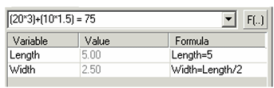You can also use this palette to assign constraints to dimensions.

# Mathematical Calculations

To perform a calculation, enter the values in the top field of the palette, using parentheses as needed to create inner expressions. No spaces are allowed. The four mathematical operators should be entered as +, -, * (multiply), and / (divide).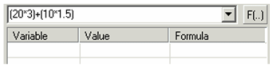To calculate the result, press Enter.You can also use these commonly-used values in place of numbers: pi =3.1415926 e =2.71828 The function (F) button enables you to perform a function on the value in the parentheses. For example, select sin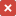to get the sine of an angle.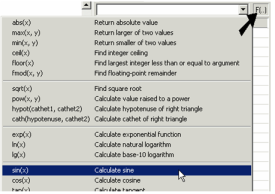sinappears in the calculator field (you also could have entered this expression yourself manually).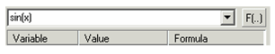Sine is calculated for radians, so if you have a value in degrees it must be converted. Look in the functions menu under Units Conversion and select rad(a), which converts degrees to radians.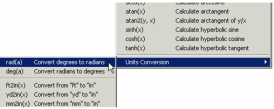Arrange the expression so that the parentheses are in the correct places, and include the angle in degrees (45 in this case):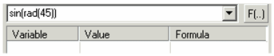Press Enter to obtain the result.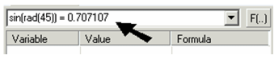Some functions require two values, such as exponentials. pow(x, y) raises x to the y power.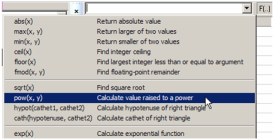Include the values and press Enter to get the result.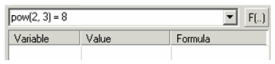# Defining and Using Variables

In addition to calculating numerical equations, you can use the Calculator Palette to define variables. Subsequent values and variables can then be based on variables you've already created. In the calculator field, enter the variable definition in the format "variable name=value" (in this example, Length=5). The value can be a number, function, or another variable. Variables are case sensitive and cannot have spaces.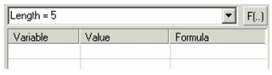Press Enter, and the variable, value, and formula are listed in the list below.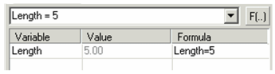You can also enter a variable and variable or formula directly in the fields of the list. The next variable can be based on an existing variable. In the calculator field, enter an equation that uses a variable name, such as "Width=Length/2."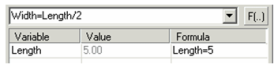Press Enter, and the new variable appears, with its calculated value.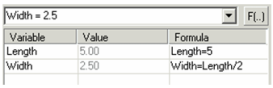# Using Expressions in Data Field

Numbers or variables from the Calculator Palette can be used as values in the Inspector Bar or Coordinate fields. As an example, define Length and Width variables, and draw a rectangle. Select the first corner point, but not the second point.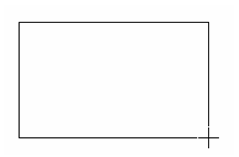Press Tab to access the first field in the Inspector Bar. The value is highlighted.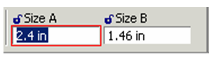Press F2 to access the Calculator Palette. In the calculator field, type Length and press Enter.Note: You could also enter a mathematical expression based on numbers, or an expression that includes one or more variables.

Back in the Inspector Bar, the first field contains the new value.For subsequent fields of the Inspector Bar or Coordinate Fields, do not press Tab to scroll. Instead, place the cursor in the desired field, then press F2 to access the Calculator Palette.